# FIN4320 Exam 4

FIN 4320 – Exam 4

1. Dan bought some shares of a company at a total cost of \$12,500 3 years ago. Today the value of these stocks is \$17,800. If Dan donates all these shares to the charity, how much would be his cash savings from this tax strategy? Assume Dan is in 25% marginal tax bracket and long term capital gain tax rate is 15%.

\$5,245

\$12,500 x 15% = \$1,875

\$17,800 x 15% = \$2,670

\$2,670 - \$1,875 = \$795

\$17,800 x 25% = \$4,450

\$4,450 + \$795 = \$5,245

2. Compute the difference between accumulated savings (net of taxes) for \$15,500 (pretax) placed in bonds yielding 6.5% in a qualified pension versus an investment in a municipal bond yielding 3.5%. The municipal bond sum deposited was made with after-tax dollars on the same pretax amount. The marginal tax rate was 25%. Assume that the sums were accumulated for 25 years and the pension was liquidated at that time.

\$28,650

Investment in a qualified pension:

Lump sum accumulated over 25 years:

N = 25

I/Y = 6.5%

PV = \$15,500

PMT = 0

FV = \$74,829.33

After-tax amount = \$74,829.33 x (1-.25) = \$56,122

Investment in a municipal bond:

Investment amount = \$15,500 x (1-.25) = \$11,625

Investment value in 25 years:

N = 25

I/Y = 3.5%

PV = \$ 11,625

PMT = 0

FV = \$27,472.72

After-tax amount = \$27,472.72

\$56,122 - \$27,472.72 = \$28,649 or \$28,650

3. Jose, age 25, currently saves \$13500 per year in his retirement account which is expected to earn 5% return. Jose is planning to retire at 62 and needs to fund his retirement upto age, 85. He has estimated that the annualamount needed during retirement would be \$47,000 in today's dollar terms. The inflation rate is expected to be 1.5%. Calculate the shortfall (if any) in his retirement account at the beginning of retirement.

No Shortfall

Part A: Find the total amount which he needs in his retirement nest to fund rest of life.

Step 1: Compute the annual requirement at the beginning of the retirement

PV = \$47,000

N = 62 – 25 = 37

I/Y = 1.5%

PMT = 0

FV = ? = \$81,534.50 = \$81,535

Step 2: Compute the real rate of return at which we need to find the present value of annual requirement of \$81,534.50 during 62 – 85.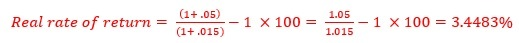Step 3: Compute the PV of annual requirement at 3.4483% for 85 – 62 = 23 years

BGN MODE

FV = 0

N = 23

I/Y = 3.4483%

PMT = \$81,535

PV = ? = \$1,324,466.63 = \$1,324,477

Part B: Calculate the additional savings if shortfall. Computer PMT. Use END MODE

PV = 0

PMT = \$13,500

N = 37

I/Y = 5%

FV = \$1,371,979.88 = \$1,371,980

\$1,371,980 - \$1,324,477 = \$47,503

No Shortfall

4. Jose, age 25, currently saves \$13500 per year in his retirement account which is expected to earn 5% return. Jose is planning to retire at 62 and needs to fund his retirement upto age, 85. He has estimated that the annualamount needed during retirement would be \$47,000 in today's dollar terms. The inflation rate is expected to be 1.5%. Calculate the lump sum amount required at the beginning of retirement to fund his post retirement period.

\$1,324,251

Part A: Find the total amount which he needs in his retirement nest to fund rest of life.

Step 1: Compute the annual requirement at the beginning of the retirement

PV = \$47,000

N = 62 – 25 = 37

I/Y = 1.5%

PMT = 0

FV = ? = \$81,534.50 = \$81,535

Step 2: Compute the real rate of return at which we need to find the present value of annual requirement of \$81,535 during 62 – 85.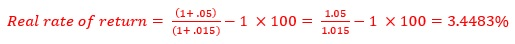Step 3: Compute the PV of annual requirement at 3.45% for 85 – 62 = 23 years

BGN MODE

FV = 0

N = 23

I/Y = 3.45%

PMT = \$81,535

PV = ? = \$1,324,259 = \$1,324,251???

5. Jose, age 25, currently saves \$13500 per year in his retirement account which is expected to earn 5% return. Jose is planning to retire at 62 and needs to fund his retirement upto age, 85. He has estimated that the annual amount needed during retirement would be \$47,000 in today's dollar terms. The inflation rate is expected to be 1.5%. Compute the additional annual savings (if needed) to fund the shortfall in retirement account (if any).

Part A: Find the total amount which he needs in his retirement nest to fund rest of life.

Step 1: Compute the annual requirement at the beginning of the retirement

PV = \$47,000

N = 62 – 25 = 37

I/Y = 1.5%

PMT = 0

FV = ? = \$81,534.50 = \$81,535

Step 2: Compute the real rate of return at which we need to find the present value of annual requirement of \$81,534.50 during 62 – 85.Step 3: Compute the PV of annual requirement at 3.4483% for 85 – 62 = 23 years

BGN MODE

FV = 0

N = 23

I/Y = 3.4483%

PMT = \$81,535

PV = ? = \$1,324,466.63 = \$1,324,477

Part B: Calculate the additional savings if shortfall. Computer PMT. Use END MODE

PV = 0

PMT = \$13,500

N = 37

I/Y = 5%

FV = \$1,371,979.88 = \$1,371,980

\$1,371,980 - \$1,324,477 = \$47,503

6. Frances bought a car for \$44,500 as a business investment opportunity. She is allowed to depreciate over 10 years and take it as business tax deduction. At the end of the 6th year she sells this car for \$22,000. Compute the cumulative cash benefit after sale on this transaction. Assume that Frances can earn 4.5% after tax returns and is in 25% tax bracket. Tax rate on gain is 15%.

\$6,842.54

Step 1: Compute the cumulative tax benefit of depreciation for 10 years

Yearly Deductible Depreciation = \$44,5000 ÷ 10 = \$4,450

Yearly Tax Benefit = \$4,450 x 25% =  \$1,125.50

N = 6

I/Y = 4.5%

PMT = \$1,112.50

PV = 0

FV = ? = \$7,472.54

Step 2: Compute the tax to be paid after 6 years on gain at the time of sale

Sale Price in the 6th year = \$22,000

Depreciated Value of the car in the 6th year =\$44,500 – (\$4,450 x 6) = \$17,800

Gain = \$22,000 - \$17,800 = \$4,200

Tax on Gain = \$4,200 x 15% = \$630

Step 3: Compute Net Cash Earned (Step 1 – Step 2)

Cash Inflow = \$7,472.54

Cash Outflow = \$630

\$7,472.54 - \$630 = \$6,842.54

Net Cash Earned = \$6,842.54

7. John made \$45,000 a year and had an annual investment cost of \$250 and fees to a tax preparer of \$525 per year. Each year John's total deduction fell short of the miscellaneous expenditure floor of 2% of AGI. He decides to cluster two years of his tax planning expenditure by visiting his tax planner twice in one year (i.e.,February for the past year and December for the next year.) How much would be deduction due to this clustering?

\$400

\$45,000 x 2% = \$900

\$250 + \$525 +\$525 = \$1,300

\$1,300 - \$900 = \$400

8. Richard and Sue wants to provide full funding for their 3 year old daughter who is expected to start college when she is 18. The current annual cost of a 4 year college is \$38,000 which is expected to increase by 3.5% per year. They expect to earn 5% on their investment. They have already saved \$8,000 in a college fund for this purpose. Calculate the additional amount they should save by the end of every year in order to accumulate funding for 4 years of college when their daughter turns 18.

\$10,780

Step 1: Annual cost of education when the child starts college

PV = \$38,000

N = 18 – 3 = 15

I/Y = 3.5%

PMT = 0

FV = ? = \$63,663.26

Step 2: Total amount required (lump sum) at the beginning of 18th year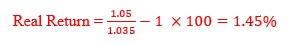BGN MODE

PMT = \$63,663.26

N = 4

I/Y = 1.45%

FV = 0

PV = ? = \$249,245.34 = \$249,245

END MODE

FV = \$249,245

I/Y = 5%

N = 15

PV = \$8,000

PMT = \$10,779.85 = \$10,780

9. Richard and Mary wants to provide full funding for their 3 year old daughter who is expected to start college when she is 18. The current annual cost of a 4 year college is \$38,000 which is expected to increase by 3.5% per year. They expect to earn 5% on their investment. Calculate the total cost (lump sum amount) required by them to fund 4 years of college when their daughter turns 18.

\$249,245

Step 1: Annual cost of education when the child starts college

PV = \$38,000

N = 18 – 3 = 15

I/Y = 3.5%

PMT = 0

FV = ? = \$63,663.26

Step 2: Total amount required (lump sum) at the beginning of 18th year

BGN MODE

PMT = \$63,663.26

N = 4

I/Y = 1.45%

FV = 0

PV = ? = \$249,245.34 = \$249,245

10. Susanna a businesswoman is in 33% tax bracket. She asks her clients to bill her in February of the following year instead of December of the current year. Her billing is for \$22,000 per year. Compute the cumulative tax benefit of this deferral if she can earn 6.25% return on her investment and plans to repeat this process for the next 15 years.

\$10,765

Yearly Tax Deferral = \$22,000 x 33% = \$7,260

Yearly Tax Benefit = \$7,260 x 6.25% = \$453.75

Cumulative Tax Benefit:

N = 15

I/Y = 6.25%

PMT = \$453.75

PV = 0

FV = ? = \$10,764.81 = \$10,765

11. A client is concerned about the impact that inflation will have on her retirement income. The client currently earns \$38,000 per year. Assuming that inflation averages 2.25% for the first five years, 2.5% for the next five years and 3.25% for the remaining time until retirement. What amount must her first-year retirement income be when she retires 13 years from now if she wants it to equal the purchasing power of her current earnings?

\$52,892

After first 5 years:

PV = \$38,000

N = 5

I/Y = 2.25%

PMT = 0

FV = ? = \$42,471.75

After the next 5 years:

PV = \$42,471.75

N = 5

I/Y = 2.5%

PMT = 0

FV = ? = \$48,052.89

Remaining years (13th year):

PV = \$48,052.89

N = 3

I/Y = 3.25%

PMT = 0

FV = \$52,891.96 = \$52,892

12. Sam and Sue wants to provide full funding for their 7 year old daughter who is expected to start college when she is 18. The current annual cost of a 4 year college is \$38,000 which is expected to increase by 3.5% per year. Sam and Sue expect to earn 4.5% on their investment. Calculate how much they should save by the end of every year in order to accumulate funding for 4 years of college when their daughter turns 18.

\$15,803

Step 1: Annual cost of education when the child starts college

PV = \$38,000

N = 18 – 7 = 11

I/Y = 3.5%

PMT = 0

FV = ? = \$55,478.85

Step 2: Total amount required (lump sum) at the beginning of 18th year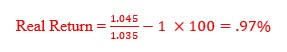Real Return =

BGN MODE

PMT = \$55,478.85

N = 4

I/Y = .97%

FV = 0

PV = ? = \$218,737.98 = \$218,738

END MODE

FV = \$218,738

I/Y = 4.5%

N = 11

PV = 0

PMT = \$15,803.42 = \$15,803

13. Jason, age 14, was given a choice of \$80,000 to be deposited in his account today orhis parents would fund 80% of lump sum cost of 4 years of college. The first year of his college cost when he turns 18 is expected to amount to exactly \$30,000 a year (i.e., in future dollar terms). Which should he take given a 7% after-tax investment return in both cases? Assume that the cost of education increases by 5% per year.

deposit, since its future value would be \$104,864 while that of 80% of cost would be \$91,597

Alternative 1: Funding 80% of college cost by parents

Step 1: Calculate the real rate (RR)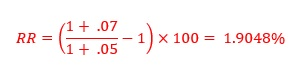Step 2: The cost of college during the 1st year is \$30,000. Calculate the total cost (lump sum) of 4 years of college as follows:

BGN MODE

N = 4

I/Y = 1.9048

PMT = \$30,000

FV = 0

PV = ? = \$116,677.18

Parents contribution = \$116,677.18 x 80% = \$93,341.74

Alternative 2: Depositing \$80,000 today. Compute future value.

BGN MODE

PV = \$80,000

I/Y = 7%

N = 4

PMT = 0

FV = \$104,863.68 = \$104,864

Depositing the \$80,000 is the better option

14. Martha has estimated that she would need \$35,000 per year (in today's \$ terms) to live on in retirement. She will be retiring in 30 years and is funding for a 23-years retirement period. The inflation rate is expected to be 2% per year and the after-tax return on her investments is expected to be 5.5%. Calculate the annual amount she would need at the beginning of her retirement.

\$63,398

Step 1: Annual amount she would need at the beginning of her retirement

PV = \$35,000

N = 30

I/Y = 2%

PMT = 0

FV = ? = \$63,697.66 = \$63,398

15. Martha has estimated that she would need \$35,000 per year (in today's \$ terms) to live on in retirement. She will be retiring in 30 years and is funding for a 23-years retirement period. The inflation rate is expected to be 2% per year and the after-tax return on her investments is expected to be 5.5%. Calculate the lump sum amount required at the beginning of her retirement.

\$1,031,578

Step 1: Cash shortfall at the beginning of retirement

PV = \$35,000

N = 30

I/Y = 2%

PMT = 0

FV = ? = \$63,697.66 = \$63,398

Step 2: Lump sum needed at the beginning of retirement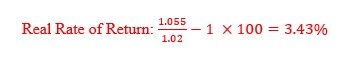Real Rate of Return:

BGN MODE

FV = 0

N = 23

I/Y = 3.43%

PMT = \$63,398

PV = ? = \$1,031.583.76  = \$1,031,578???

16. Victor bought a car for \$42,500 as a business investment opportunity. He is allowed to depreciate over 10 years and take it as business tax deduction. At the end of the 10th year he sells this car for \$22,000. Compute the cumulative cash benefit after sale on this transaction. Assume that Victor can earn 5% after tax returns and is in 33% tax bracket. Tax rate for Victor on gain is 15%.

\$14,340

Step 1: Compute the cumulative tax benefit of depreciation for 10 years

Yearly Deductible Depreciation = \$42,500 ÷ 10 = \$4,250

Yearly Tax Benefit = \$4,250 x 33% =  \$1,402.50

N = 10

I/Y = 5%

PMT = \$1,402.50

PV = 0

FV = ? = \$17,640.49

Step 2: Compute the tax to be paid after 10 years on gain at the time of sale

Sale Price in the 10th year = \$22,000

Depreciated Value of the car in the 6th year =\$42,500 – (\$4,250 x 10) = \$0

Gain = \$22,000 - \$0 = \$22,000

Tax on Gain = \$22,000 x 15% = \$3,300

Step 3: Compute Net Cash Earned (Step 1 – Step 2)

Cash Inflow = \$17,640.49

Cash Outflow = \$3,300

\$17,640.49 - \$3,300 = \$14,340.49

Net Cash Earned = \$14,340.49 = \$14,340